# Mathematics 1010 online

## Worked Word Problems

This page contains a number of worked word problems. Of course, no list could be complete, but there are certain pattern that occur over and over.

1. Percent, unknown base amount. After a 10 percent raise your hourly wage is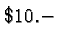. How much did you make before?

Solution: Let's say your previous wage was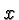dollars per hour. You are now making 10 percent more. In other words, you are making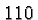% of your old wage. Hence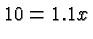which means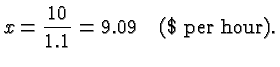2. Percent, unknown discount and tax. You buy a pair of pants whose listed price is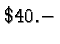. There is a sale, and the dealer offers a discount of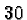%. However, you also have to pay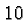% sales tax. What do you pay in total?

Solution: The discount applies to the list price of. The sales tax applies to the discounted price.% ofis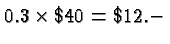. The discounted price therefore is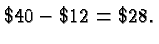(Equivalently you could argue that you buy the pants for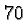% of the list price, which is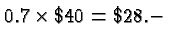) You still need to pay% sales tax on the price of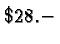. Since% percent ofis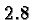your total price is3. Percent, unknown percentage. Ten years ago, the population of your town was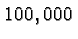. Now it is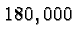. What is the average annual growth rate?

Solution: Let's call the growth rate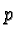. Every year the population gets multiplied with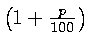. The problem tells us that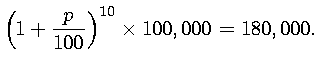Dividing byon both sides gives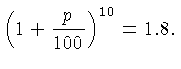To get rid of the exponentwe take both sides to the power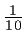. (See the pages on powers and roots and radicals. ) This gives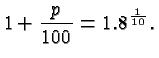Subtracting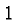on both sides, and then multiplying with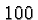gives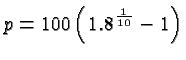This expression can be evaluated with a calculator, and works out to approximately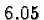%.

4. Mixture Problems. Suppose you mix a gallon of 5% vinegar with two gallons of 20%vinegar. What's the percentage of vinegar in the resulting mixture?

Solution: The main difficulty here is to understand the meaning of the phrase 5% vinegar. Think of ordinary vinegar as a mixture of water and pure (100%) vinegar. 5% vinegar means that the ratio of the pure vinegar in the mixture, and the total amount of fluid is 0.05 (or 5%). In this example the total amount of the fluid is 3 gallons, and the total amount of pure vinegar is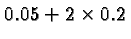gallons (i.e., 5% of the 1 gallon and 20% of the two gallons). The ratio of pure vinegar to total amount of fluid is, therefore,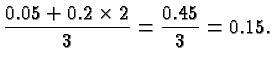The result is 15% vinegar. In variations of this problem other ingredients may be unknown (like the amount you add to get a mixture of a specified strength.) In that case use a variable for the unknown, and solve the resulting equation.

5. Work Rate Problems. You can paint a room in 2 hours, and your brother can do the same in 3 hours. How long does it take the two of you working together?

Solution: The trick for this kind of problem is to think of the job as the basic unit. You want to do 1 job, i.e., paint that room. You can do 1/2 of that job in one hour, and your brother can do 1/3 of the job in one hour. Painting together the two of you will do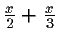of that job inhours. Thus we need to solve the equation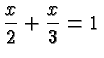which gives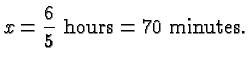There are variations of this. depending on what is known, but in all cases think of the job as your basic object.

6. Pythagorean Theorem Problems. Your laser range meter tells you that the distance to the top of a nearby mountain is 3 miles. Your map tells you that the horizontal distance from you to (a point underneath) the top of that mountain is 2.8 miles. How tall is the mountain?

Solution: The solution to all such problems proceeds by drawing a picture, and then applying the Pythagorean Theorem. In this case we get the following Figure which shows the mountain in a fetching green color: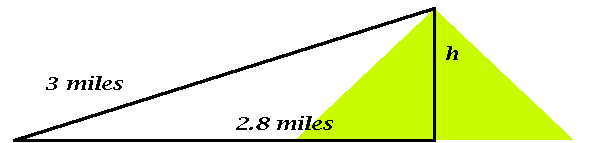Denoting the unknown height by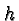we get the equation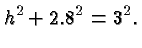Subtracting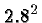and taking the square root on both sides gives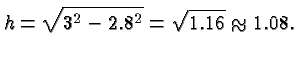That mountain is a little over a mile high (like some of the mountains near Salt Lake City).

7. Scaling Problems You cover the replica of a famous statue of Archimedes with gold leaf, and you pay $200 for the gold. You like the statue so much that your spouse acquires the original, and gives it to you for your birthday. The original is just like the replica, except it's 3 times as tall, wide, and deep. Both the replica and the original are made of solid marble. You cover the original statue with gold leaf also. How much do you pay for the required gold? Your replica weighs 2 tons. How much does the original statue weigh? Solution: There are quite a few problems like this in the homework sets because they provide excellent exercises in applying radicals. As pointed out on the scaling page, multiplying each linear dimension with a factor multiplies every area with the square of that factor, and the volume (and weight) with the cube of that factor. Everything flows from that basic fact! In this case we multiply linear dimensions with 3, and so we multiply the area (and hence the price of the gold leaf) with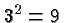and the volume (and hence the weight) of the statue with. You pay$1,800 for the gold leaf, and your original statue weights 54 tons.

You now begin to wonder how much it will cost to reinforce your floor, or whether you should perhaps remove the floor altogether and place your statue in the basement. But it is a beautiful statue!

In some of the homework problems in this course you are given (explicitly or implicitly) a factor that multiplies the area or the volume. As explained on the scaling page, if you multiply the areas with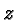you multiply the lengths with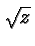and the volume with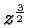, and if you multiply the volume withyou multiply the lengths with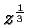and the areas with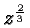.

8. Throwing Rocks.

You throw a rock and release it at a height of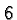feet. Its initial vertical velocity is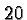feet per second (going up) and its horizontal (forward) velocity is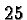feet per second. How far does the rock fly until it hits the ground, and what is the maximum height that it reaches?

Solution: According to the discussion of throwing rocks the height of the rock at the time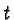is given in general byIn our case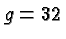feet per second squared (since we are on earth),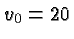feet per second (the initial upward motion), and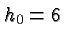feet, the height at which we release the rock. The height of the rock at time, therefore, is given by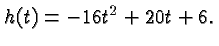To find out at what time the rock hits the ground (where the height is zero) we need to solve the quadratic equation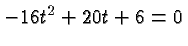which gives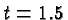seconds as the time of impact. During that time the rock covers a distance that's obtained by multiplying the flight time with the horizontal velocity, which gives the distance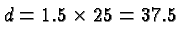feet. At the time that the rock reaches its maximum height its vertical velocity, which in general is given by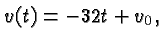is zero. In our caseand hence the time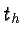at which the rock reaches the maximum height is given by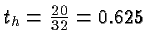seconds. At that time the rock is a height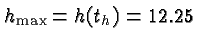feet.